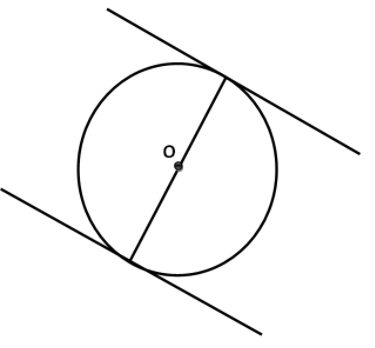A circle have a parallel tangents at most _____.

# A circle have a parallel tangents at most _____.

1. A
Two
2. B
Four
3. C
Five
4. D
Three

Fill Out the Form for Expert Academic Guidance!l

+91

Live ClassesBooksTest SeriesSelf Learning

Verify OTP Code (required)

### Solution:

A line that touches a circle at a single point is called a tangent to the circle.
A circle can have at most 2 parallel tangents, one at a point on it and one at a point diametrically opposite from it.Hence, Option (1) is the correct answer.

## Related content

 Area of Square Area of Isosceles Triangle Pythagoras Theorem Triangle Formula Perimeter of Triangle Formula Area Formulae Volume of Cone Formula Matrices and Determinants_mathematics Critical Points Solved Examples Type of relations_mathematics+91

Live ClassesBooksTest SeriesSelf Learning

Verify OTP Code (required)